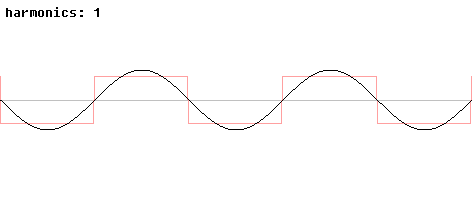# Explain THD & TDD in power quality.

The total harmonic distortion, or THD, of a signal is a measurement of the harmonic distortion present and is defined as the ratio of the sum of the powers of all harmonic components to the power of the Fundamental frequency.TDD(I) = Total Current Demand Distortion is Calculated harmonic current distortion against the full load (demand) level of the electrical system. At the full load TDD(I)=THD(I). So TDD gives us better insight about how big impact of harmonic distortion in our system. For example we could have very high THD but the load of the system is low. In this case the impact on the system is also low.